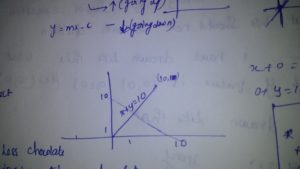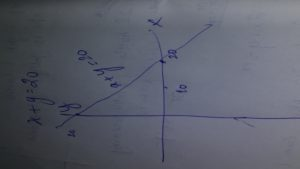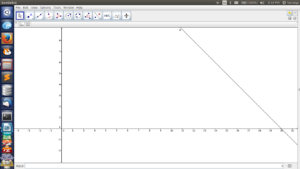# Linear Equation with story

saranya

We have started with the story of linear equation. Linear equation is nothing but a straight line and choice. For an example we have taken x+y=10. The story of the linear equation is, I and my brother likes chocolates if I get more chocolate obviously he is going to get less chocolate that is the story of the linear equation. After that we have entered into the problem. We started drawing the line for that equation.First I have drawn wrongly for that equation x+y=10.What I have Thought is when the x increases y also get increase equally so the point is (10,10) that is why I have drawn like that.  After that I have understood the function of linear equation. In that linear equation it will get increases by 10 on both the axis.

When I learned how to draw a linear equation. I have made my own question that is x+y=20. Then I have checked my equation using geogebra.